## K-means/medoids clustering

In a k-means or medoids clustering, features are clustered into k separate clusters. The procedures seek to find an assignment of features to clusters, for which the distances between features within the cluster is small, while distances between clusters are large.

Toolbox | Transcriptomics Analysis ()| Feature Clustering | K-means/medoids Clustering (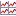)

Select at least two samples ( () or ()) or an experiment ().

Note! If your data contains many features, the clustering will take very long time and could make your computer unresponsive. It is recommended to perform this analysis on a subset of the data (which also makes it easier to make sense of the clustering). See how to create a sub-experiment in Creating sub-experiment from selection.

Clicking Next will display a dialog as shown in figure 28.92.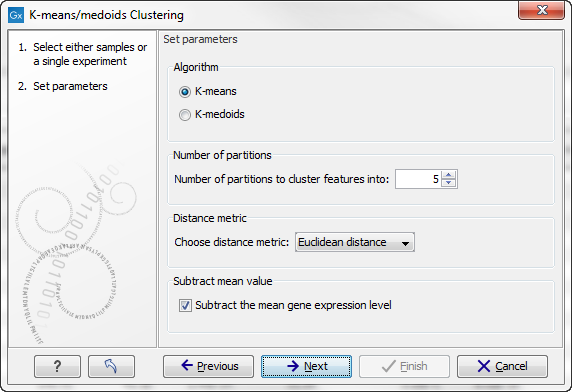Figure 28.92: Parameters for k-means/medoids clustering.

The parameters are:

• Algorithm. You can choose between two clustering methods:
• K-means. K-means clustering assigns each point to the cluster whose center is nearest. The center/centroid of a cluster is defined as the average of all points in the cluster. If a data set has three dimensions and the cluster has two points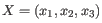and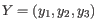, then the centroid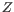becomes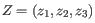, where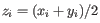for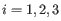. The algorithm attempts to minimize the intra-cluster variance defined by: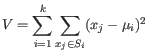where there are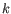clusters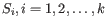and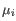is the centroid of all points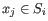. The detailed algorithm can be found in [Lloyd, 1982].
• K-medoids. K-medoids clustering is computed using the PAM-algorithm (PAM is short for Partitioning Around Medoids). It chooses datapoints as centers in contrast to the K-means algorithm. The PAM-algorithm is based on the search forrepresentatives (called medoids) among all elements of the dataset. When having foundrepresentativesclusters are now generated by assigning each element to its nearest medoid. The algorithm first looks for a good initial set of medoids (the BUILD phase). Then it finds a local minimum for the objective function: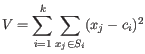where there areclustersand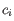is the medoid of. This solution implies that there is no single switch of an object with a medoid that will decrease the objective (this is called the SWAP phase). The PAM-agorithm is described in [Kaufman and Rousseeuw, 1990].
• Number of partitions. The number of partitions to cluster features into.
• Distance metric. The metric to compute distance between data points.
• Euclidean distance. The ordinary distance between two elements - the length of the segment connecting them. If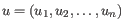and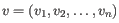, then the Euclidean distance between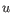and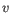is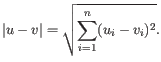• Manhattan distance. The Manhattan distance between two elements is the distance measured along axes at right angles. Ifand, then the Manhattan distance betweenandis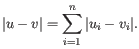• Subtract mean value. For each gene, subtract the mean gene expression value over all input samples.

Clicking Next will display a dialog as shown in figure 28.93.Figure 28.93: Parameters for k-means/medoids clustering.

At the top, you can choose the Level to use. Choosing 'sample values' means that distances will be calculated using all the individual values of the samples. When 'group means' are chosen, distances are calculated using the group means.

At the bottom, you can select which values to cluster (see Selecting transformed and normalized values for analysis).

Click Next if you wish to adjust how to handle the results. If not, click Finish.

Subsections